# Edexcel A Level Economics A:复习笔记2.4.3 Equilibrium Levels of Real National Output

### Real National Output Equilibrium

#### Short-run Equilibrium

• Real national output equilibrium occurs where aggregate demand intersects with aggregate supply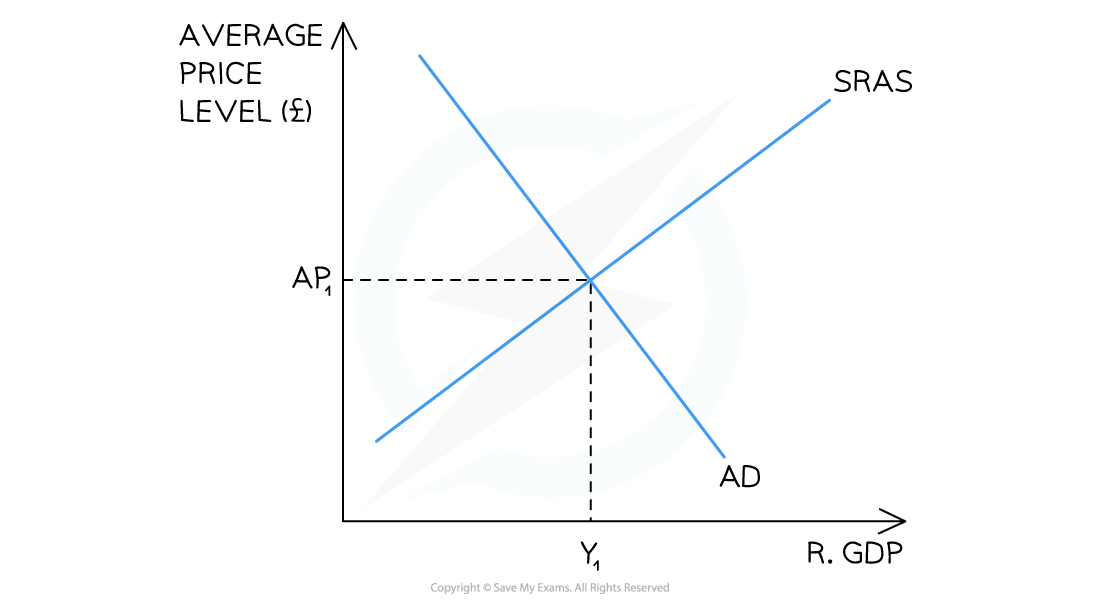A diagram showing the Classical short-run equilibrium in an economy resulting in an equilibrium price of AP1 and real output of Y1

• According to classical theory, this economy is in short run equilibrium at AP1Y1
• Any changes to the components of AD will cause the AD curve to shift left or right creating a new short-run equilibrium
• Any changes to the determinants of SRAS will shift the SRAS curve left or right creating a new short-run equilibrium

#### Long-run Equilibrium

• Classical and Keynesian economists have different views on the long-run equilibrium of real national output
• Classical economists believe that the economy will always return to its full potential level of output and all that will change in the long-run, is the average price level
• Keynesian economists believe that the economy can be in long-run equilibrium at any level of outputA diagram that shows the Classical view of long-run equilibrium which occurs at the intersection of long-run aggregate supply (LRAS), short-run aggregate supply (SRAS) & aggregate demand (AD)

Diagram Analysis

• The LRAS curve demonstrates the maximum possible output of an economy using all of its scarce resources
• The SRAS intersects with AD at the LRAS curve
• This economy is producing at the full employment level of output (YFE)
• The average price level at YFE is AP1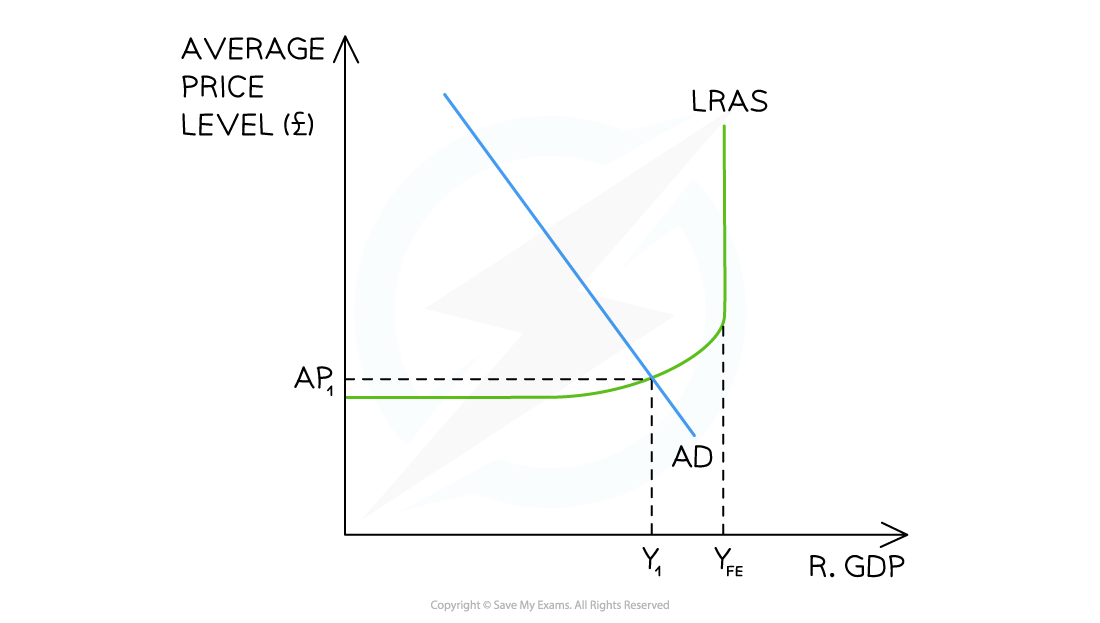A diagram that shows the Keynesian view of long-run equilibrium which occurs at the intersection of long-run aggregate supply (LRAS) & aggregate demand (AD)

Diagram Analysis

• The vertical portion of the LRAS curve corresponds to the classical view of LRAS
• The Keynesian view believes there is a maximum level of possible output
• The LRAS curve becomes elastic at a certain price level as prices cannot fall further
• Possibly due to minimum wage laws, the existence of trade unions, or long-term employment contracts preventing wage decreases
• Real output national equilibrium can occur at any level of output
• In this case equilibrium is at the intersection of LRAS and AD (AP1Y1)

### Changes in the Equilibrium Price Level & Real National Output

#### Changes Using the Classical Approach

1. An Increase in Aggregate Demand (AD)A diagram showing the Classical representation of an increase in aggregate demand (AD)

Diagram Analysis

• The initial equilibrium level of output was at AP1Y1
• Average prices in the economy rise to AP2 and the real level of output increases to Y2
• The new short-run equilibrium is at AP2Y2

2. An increase in short run aggregate supply (SRAS)A diagram showing the Classical representation of an increase in the short-run aggregate supply (SRAS)

Diagram Analysis

• The initial equilibrium level of output was at AP1Y1
• This equilibrium represents a recessionary or negative output gap equal to Y1YFE
• An increase in one of the determinants of SRAS (e.g. productivity) causes the SRAS to increase SRAS1→SRAS2
• Average prices in the economy fall to AP2 and the real level of output increases to Y2
• The new short-run equilibrium is at AP2Y2
• There is still a negative output gap but it is smaller (Y2YFE)

#### Changes Using the Keynesian Approach

1. Changes to Aggregate Demand (AD)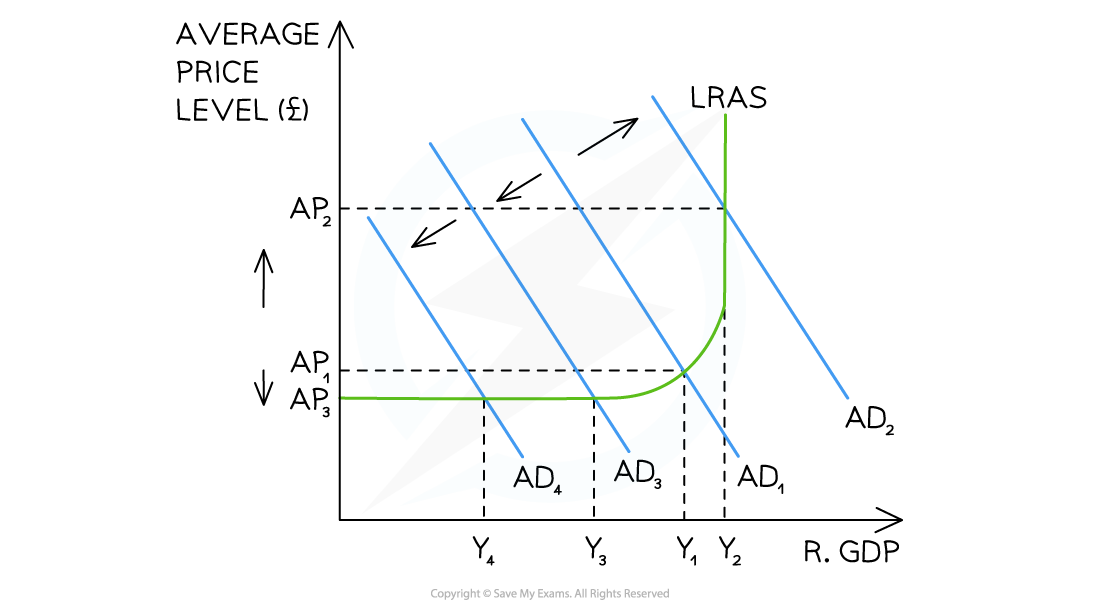A diagram that illustrates the Keynesian view of how changes to aggregate demand (AD) have different impacts on the average price level (AP)

Diagram Analysis

• The economy is initially in equilibrium at the intersection of AD1 and LRAS
• The price level is AP1 and the output is at Y1
• As this increase occurs close to the full employment level of output, there is a large increase in the average price level (AP1→AP2), but a relatively small increase in real output (Y1→Y2)
• As this decrease occurs close to the elastic portion of the LRAS curve, there is a relatively larger decrease in the real output (Y1→Y3) than the decrease in the average price level (AP1→AP3)
• If a further decrease in AD were to take place from AD3→AD4, there would be virtually no impact on the average price level, but the real output would fall from Y3→Y4

#### Exam Tip

One of the key differences that you are examined on with regard to the Classical and Keynesian models, is the understanding that a change to aggregate demand (AD) potentially has a different impact on the economy under the Keynesian model. A change to AD in the Classical model will always increase or decrease the average price level. A change to AD in the Keynesian model may not change the price level at all. This is due to the elastic portion of the LRAS curve in the Keynesian model.

#### Changes to Long-run Aggregate Supply (LRAS)

1. Changes to LRAS in the Classical Model

• Changes to any of the determinants of LRAS will change the long-run productive potential of the economy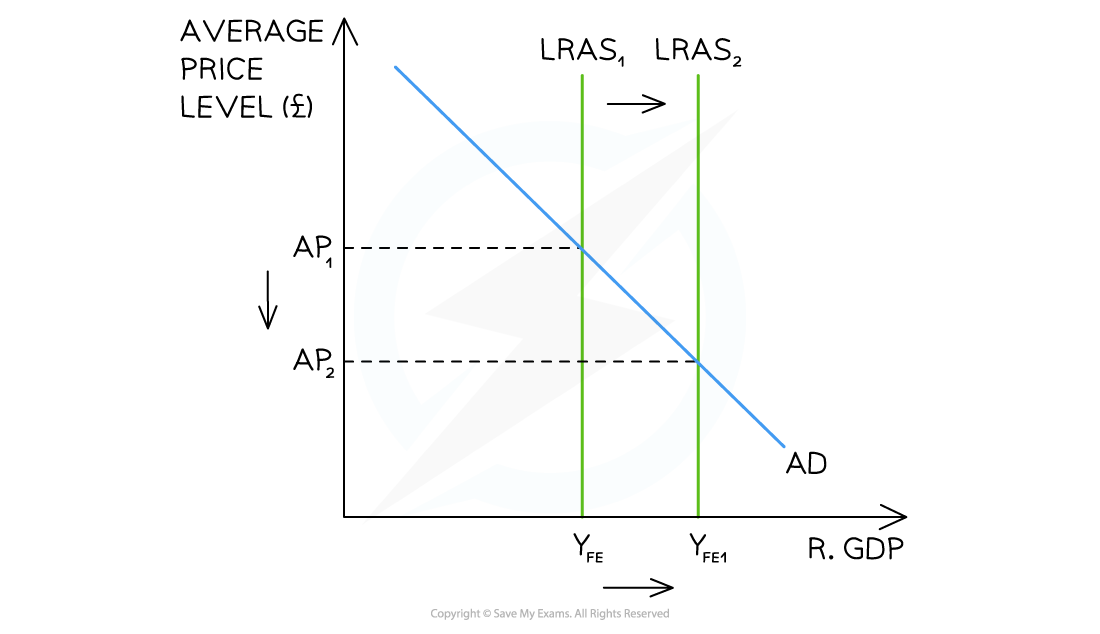A diagram showing the Classical view of an increase in the long-run aggregate supply (LRAS) of an economy and how it lowers average price levels

Diagram Analysis

• The initial potential output of this economy is seen at YFE
• The economy is in equilibrium at AP1YFE
• A change to the education level in the economy can increase the quality of labour and shift the LRAS to the right from LRAS1→LRAS2
• There is now an increased level of possible output in the economy YFE1
• The extra supply in the economy allows prices to fall and output to increase resulting in a new equilibrium at AP2YFE1

2. Changes to LRAS in the Keynesian Model

• As with the Classical model, changes to any of the determinants of LRAS will change the long-run productive potential of the economy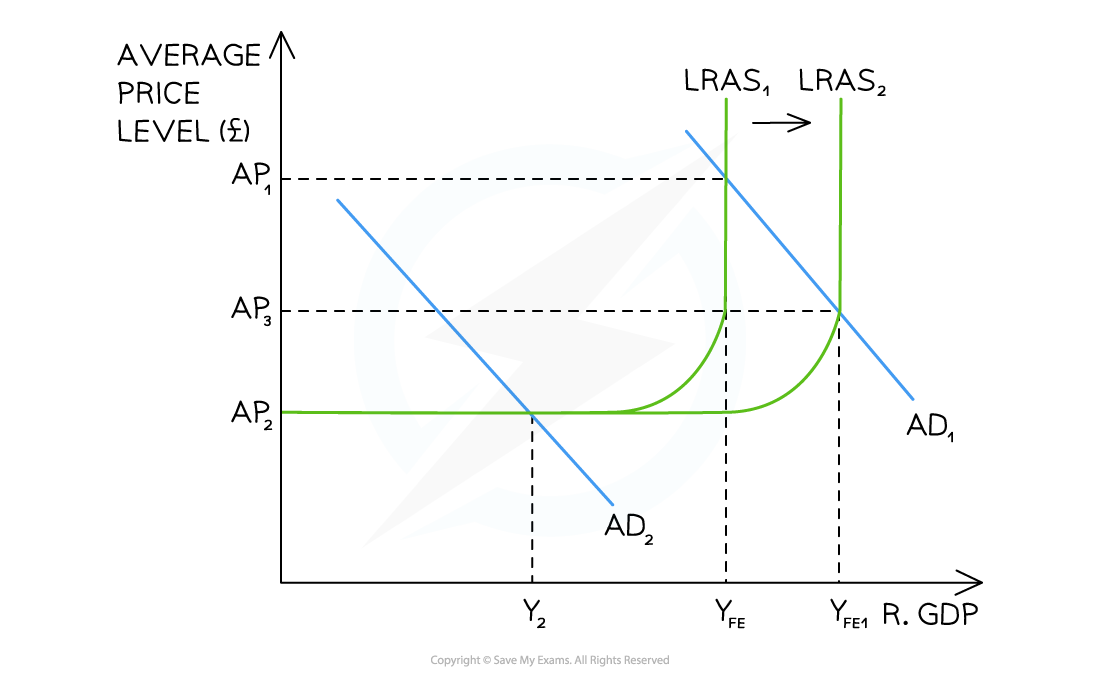A diagram showing the Keynesian view of an increase in the long-run aggregate supply (LRAS) of an economy and how it changes output without necessarily changing average price levels

Diagram Analysis

• The initial potential output of this economy is seen at YFE
• The economy is in equilibrium at the intersection of AD1 and LRAS (AP1YFE)
• A change to the immigration policy can increase the quantity of labour and shift the LRAS to the right from LRAS1→LRAS2
• There is now an increased level of possible output in the economy YFE1
• AD is in the vertical portion of the LRAS curve so output will increase (YFE→YFE1) and average prices will fall (AP1→AP3)
• If the starting point of this economy had been the equilibrium at AD2Y2, then according to Keynesian thinking, an increase in LRAS would not impact the economy as it is stuck in a depression & requires AD to increase in order to change national output